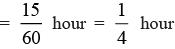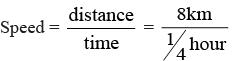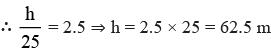Courses

# Olympiad Test: High Order Thinking Skills -1

## 20 Questions MCQ Test Science Olympiad Class 7 | Olympiad Test: High Order Thinking Skills -1

Description
This mock test of Olympiad Test: High Order Thinking Skills -1 for Class 7 helps you for every Class 7 entrance exam. This contains 20 Multiple Choice Questions for Class 7 Olympiad Test: High Order Thinking Skills -1 (mcq) to study with solutions a complete question bank. The solved questions answers in this Olympiad Test: High Order Thinking Skills -1 quiz give you a good mix of easy questions and tough questions. Class 7 students definitely take this Olympiad Test: High Order Thinking Skills -1 exercise for a better result in the exam. You can find other Olympiad Test: High Order Thinking Skills -1 extra questions, long questions & short questions for Class 7 on EduRev as well by searching above.
QUESTION: 1

### Which of the following graphs does not represent uniform speed?

Solution:

In a uniform speed, the change in position of the body remains the same for a particular interval of time. The distance travelled in option (a), (c) and (d) is the same, in (c) it is zero. But the distance changes in option (b). Hence, the answer is (b).

QUESTION: 2

### With what speed should a car travel so that it can cover a distance of 8 km. in 15 minutes?

Solution:

Time  = 15 minutesdistance = 8 km= 8 × 4 km/hour
= 32 km/h

QUESTION: 3

### An odometer is used to measure

Solution:
QUESTION: 4

In the Celsius scale, the upper fixed point is

Solution:

The upper fixed point in the Celsius scale is 100°C, which is the boiling point of water.

QUESTION: 5

When same amount of heat is supplied to hydrogen peroxide, iron, and carbon dioxide, which substance expands the most?

Solution:

Gases  expand the most upon heating because of the large intermolecular distances and less intermolecular force. Carbon dioxide being a gas will expand the most.

QUESTION: 6

Conduction cannot take place in

Solution:

A medium (solid) is required for conduction to take place.

QUESTION: 7

The speed of light is

Solution:

The speed of light decreases with an increase in the density of the medium.

QUESTION: 8

At a particular time, the constant of ratio of length of a light pole and the length of its shadow is found to be 2.5 m. Now, the length of the shadow of a flag pole is 25 m, calculate the length of the pole.

Solution:

Let the height of the flag pole be ‘h’ The length of the light pole = 25 m The constant ratio of length of object to its shadow = 2.5 (given)QUESTION: 9

We can create enlarged, virtual images with

Solution:
QUESTION: 10

In which circuit will the bulb (s) glow the brightest?

Solution:

The chemical energy stored in two cells is more than in one cell. The electrical energy used by one bulb is less than the energy used by two bulbs. So, the bulb in the circuit that has one bulb and two cells will glow the brightest.

QUESTION: 11

An electric heater is used in kitchens to cook food, it converts electrical energy to

Solution:

A heater is used to obtain heat energy. For light energy, a bulb or other sources are used.

QUESTION: 12

Which of the following is not a chemical change?

Solution:

Here, only a change of state from liquid and solid to gas is seen, and no new substances are formed

QUESTION: 13

When you put a candle in a vessel and heat the vessel, you will observe that the candle does not burn, it changes its shape and state. What kind of change is this?

Solution:

The candle started melting, but since no new substance was formed, it is a physical change.

QUESTION: 14

The metal which can displace hydrogen from acid is

Solution:

Calcium is more reactive than hydrogen.

QUESTION: 15

Na2 CO3+ 2HC1 ?

Solution:
QUESTION: 16

The metal which can displace zinc from its solution is

Solution:

Magnesium is more reactive than zinc.

QUESTION: 17

When a vehicle moves very fast, _________ is created temporarily behind it.

Solution:

When a wind flows very fast, a low pressure is produced temporarily which is filled by the rushing wind nearby.

QUESTION: 18

Sea and land breezes are caused due to

Solution:

Sea and land breezes are caused because of an uneven heating of the earth and through convection currents.

QUESTION: 19

When water is cooled, it contracts until it reaches ______ and then it starts expanding.

Solution:

Though substances contract on cooling. water expand when cooled beyond 4°C. This is known as anomalous behaviour of water.

QUESTION: 20

Our body temperature is maintained at

Solution:

Human beings are warm blooded, which means that their body temperature has to be maintained around a particular temperature which is 37°C or 98° F. Water plays an important role in this.